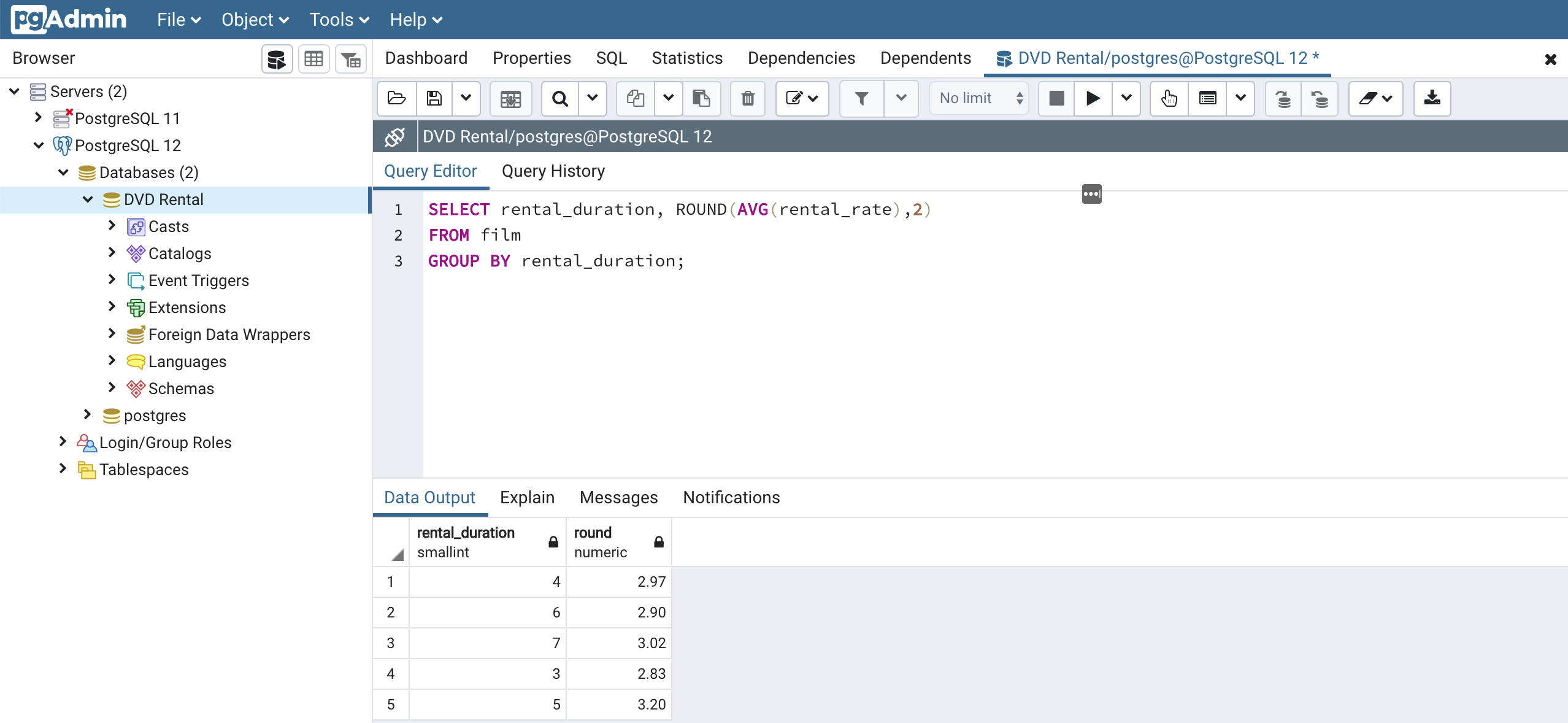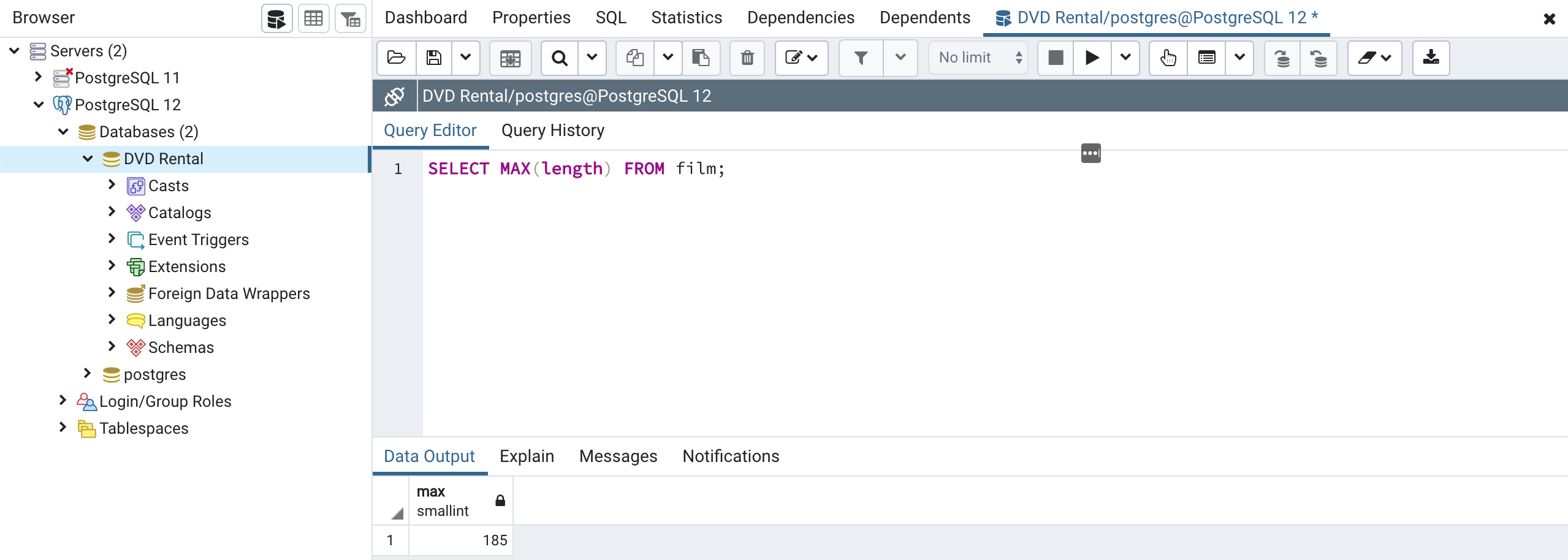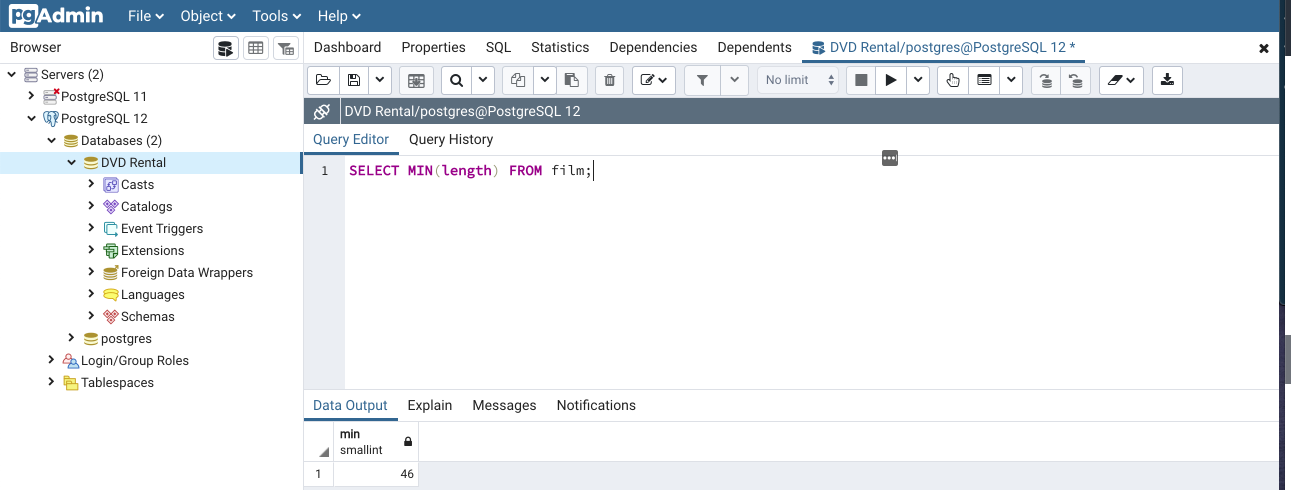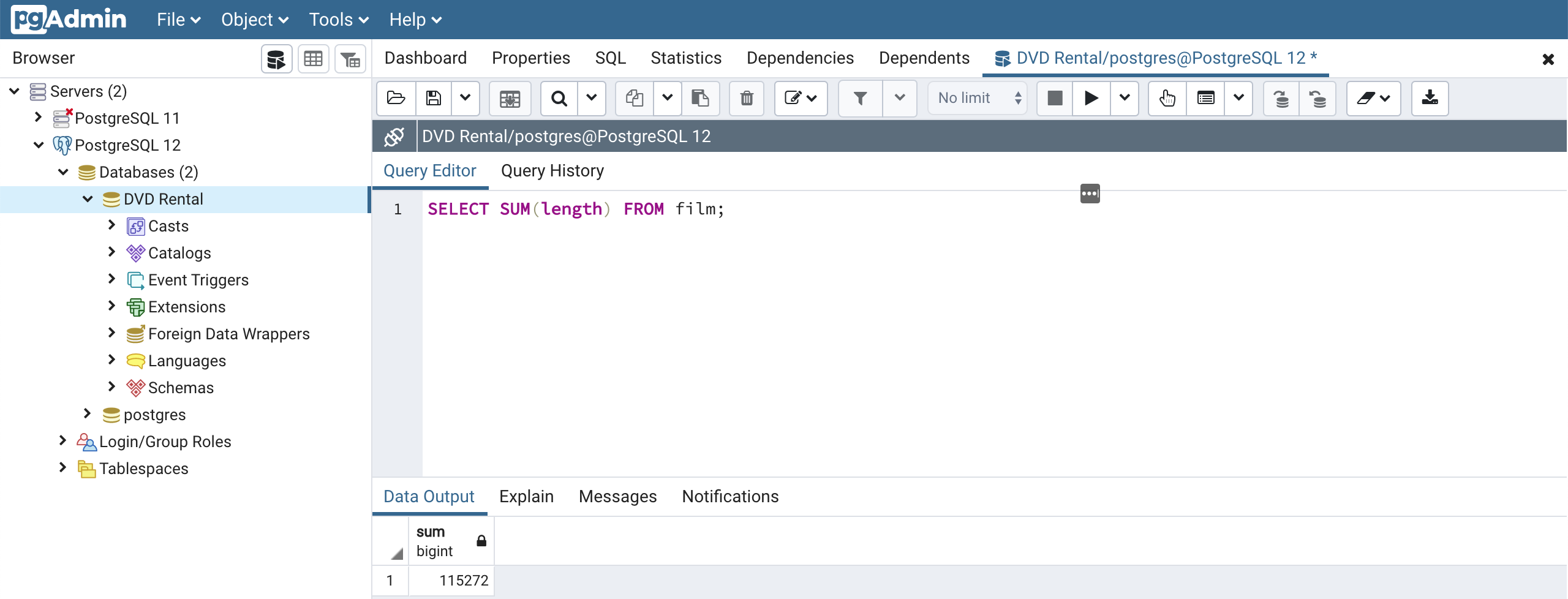# How To Write SQL GROUP BY Statements

Hey - Nick here! This page is a free excerpt from my \$99 course SQL Fundamentals.

I have a 30-day satisfaction guarantee, so there's no risk (and a ton of upside!) in signing up for this course and leveling up your SQL skills today!

In this tutorial, you will learn how to use the SQL GROUP BY statement ot group your data into buckets according to their value in a specific column. You will also learn you to use aggregate functions to perform calculations on database data based on the data's category iin the GROUP BY statement.

GROUP BY statements are one of the most important concepts for SQL programmers to understand. They are also fairly complex. Accordingly, be sure to understand the concepts from this lesson before proceeding through this course.

## What is a SQL GROUP BY Statement?

The SQL GROUP BY statement allows us to easily aggregate columns into categories. Let's explore this idea with a simple example from our DVD Rental database.

We saw in our last lecture that within the `film` table, there is a column called `rental_rate` that contains the rental prices for each movie in the table. The `rental-rate` column only holds three different values:

• \$0.99
• \$2.99
• \$4.99

We can treat each of these rental rates as its own category using a GROUP BY statement. Once we have used the GROUP BY statement to create categories, we use an aggregate function to perform calculations on the values within those categories.

Let's consider a generalized example. Here is the syntax for a typical GROUP BY statement:

``````SELECT category_column, AGG(data_column)

FROM table_name

GROUP BY category_column;``````

In this SQL example, `category_column` is the column that we are using to define our categories. `data_column` is the column that we are performing aggregate functions on, while AGG is a hypothetical aggregate function. `table_name` is the table that contains both `category_column` and `data_column`.

It is important to note that a GROUP BY clause must appear right after a FROM statement or a WHERE statement. If this is not the case, your query will return an error.

This will make more sense once we have actually learned some aggregate functions. Let's explore SQL aggregate functions next.

## What Are Aggregate Functions?

You cannot use the SQL GROUP BY statement without pairing it with an aggregate function. With that in mind, let's learn what aggregate functions are and how they interact with the GROUP BY clause.

The purpose of aggregate functions is to take multiple inputs and return a single output.

PostgreSQL includes a number of aggregate functions. You can read the PostgreSQL documentation for a complete list of aggregate functions

In this section, I will introduce some of the most important aggregate functions and provide examples of how they function using our DVD Rental database. Specifically, I will introduce the following functions:

• AVG
• COUNT
• MAX
• MIN
• SUM

## The SQL AVG Function

The SQL AVG function allows you to easily calculate the average value of section of values.

For example, here is how you would calculate the average value of the `rental_rate` column from the `film` table in our DVD Rental database:

``````SELECT AVG(rental_rate)

FROM film;``````

Here is what the output of this SQL query looks like in pgAdmin:As you can see, the average value in the `rental_rate` column is \$2.98.

We can pair the AVG function with the GROUP BY clause to generate average values within categories.

As an example, consider the `rental_duration` column within the `film` table. This column contains integer values from 3 to 7 that describe how long a customer can rent a specific movie for.

Let's write a SQL query using GROUP BY that calculates the average value of `rental_rate` for movies in each `rental_duration` category:

``````SELECT rental_duration, ROUND(AVG(rental_rate),2)

FROM film

GROUP BY rental_duration;``````

Note that I have also wrapped the AVG function in a ROUND function with a second argument of `2` to make the output of the query more readable.

Here's what the output of this query looks like:For the other aggregate functions that we explore in this lesson, we will follow a similar recipe of categorizing movies into different buckets based on `rental_duration`, and then applying aggregate functions to those categories.

### The SQL COUNT Function

The SQL COUNT function combined with the GROUP BY clause allows you to easily count the number of items contained in each category.

We have already worked with the SQL COUNT function earlier in this course to count all of the rows within a specific column. However, we have not yet paired it with the GROUP BY statement, which has limited its capability.

The COUNT function simply counts the number of rows returned by a given SQL query. This means it does not matter which column the COUNT method is applied to. By convention, it is considered a best practice to simply use `COUNT(*)` in any SQL query.

Let's look at an example where we categorize the rows of the `film` table based on `rental_duration` and count the number of rows in each category:

``````SELECT rental_duration, COUNT(*)

FROM film

GROUP BY rental_duration;``````

Here is the output of this SQL query:### The SQL MAX Function

The SQL MAX function allows you to easily determine the maximum value of a column.

As an example, we could determine the maximum value of the `length` column of the `film` table with the following SQL query:

``SELECT MAX(length) FROM film;``

Here is the output of this query:As you can see, the film with the maximum length is 185 minutes long.

The SQL MAX function can be used with GROUP BY to determine the maximum values of different categories. Let's again divide our `film` table into categories based on `rental_duration` and try and find the longest film within each category.

We can answer this question with the following query:

``````SELECT rental_duration, MAX(length)

FROM film

GROUP BY rental_duration;``````

The output of this code is below:### The SQL MIN Function

Like the SQL MAX function, the SQL MIN Function allows you easily find the smallest value within a table column.

As an example, we can find the shortest movie in the `film` table with the following query:

``SELECT MIN(length) FROM film;``As you can see, the shortest film in our DVD Rental database is 46 minutes long.

Let's explore how we can pair the SQL MIN function with the GROUP BY clause by finding the shortest film in each `rental_duration` category. The following query would answer this question for us:

``````SELECT rental_duration, MIN(length)

FROM film

GROUP BY rental_duration;``````

Here is the output of this query:### The SQL SUM Function

The SQL SUM function performs similarly to the other aggregate functions explored in this lesson. Namely, it allows us to calculate the sum of either a column (when applied to an entire column) or a category (when combined with the GROUP BY clause).

Here is an example of how we could use the SUM function to calculate how long it would take to watch every movie in the DVD Rental database:

``SELECT SUM(length) FROM film;``

Here is the output of this query:As you can surely imagine by now, we can also combine the SUM function with the GROUP BY clause to calculate the sum of different categories within our table.

Here is how you could calculate the sum of the `length` column for every `rental_duration` category in a SQL query:

``````SELECT rental_duration, SUM(length)

FROM film

GROUP BY rental_duration;``````

Here is the output of this code:## Final Thoughts

In this lesson, we explored how to use aggregate functions to take in multiple values, perform a calculation, and return a single value. Specifically, we worked with the following aggregate functions:

• AVG
• COUNT
• MAX
• MIN
• SUM

In the next section of this course, we'll explore the SQL HAVING keyword in detail.